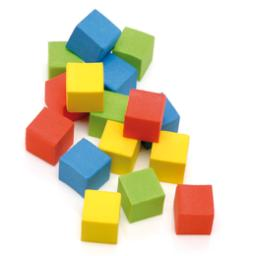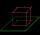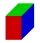# Body diagonal

Find the cube surface if its body diagonal has a size of 6 cm.

S =  72 cm2

### Step-by-step explanation:Did you find an error or inaccuracy? Feel free to write us. Thank you!Tips to related online calculators
Pythagorean theorem is the base for the right triangle calculator.

#### You need to know the following knowledge to solve this word math problem:

We encourage you to watch this tutorial video on this math problem:

## Related math problems and questions:

• Body diagonal - cubeCalculate the surface and cube volume with body diagonal 15 cm long.
• Cube diagonalsDetermine the volume and surface area of the cube if you know the length of the body diagonal u = 216 cm.
• Body diagonalCalculate the length of the body diagonal of the 6cm cube.
• Space diagonalThe space diagonal of a cube is 129.91 mm. Find the lateral area, surface area and the volume of the cube.
• Center of the cubeThe Center of the cube has a distance 16 cm from each vertex. Calculate the volume V and surface area S of the cube.
• Body diagonalCalculate the cube volume, whose body diagonal size is 75 dm. Draw a picture and highlight the body diagonal.
• Cube wallThe perimeter of one cube wall is 120 meters. Calculate the surface area and the body diagonal of this cube.
• Surface and volume - cubeFind the surface and volume of a cube whose wall diagonal is 5 cm long.
• Cube diagonalsThe cube has a wall area of 81 cm square. Calculate the length of its edge, wall, and body diagonal.
• Body diagonalCalculate the volume and surface of the cube if the body diagonal measures 10 dm.
• Cube cutThe cube ABCDA'B'C'D ' has an edge of 12cm. Calculate the area of diagonal cut B DD'B '.
• Body diagonalFind the length of the body diagonal of a cuboid with lengths of 16 cm, 7 cm, and 4 cm.
• Nice prismCalculate the cuboid's surface if the sum of its edges is a + b + c = 19 cm and the body diagonal size u = 13 cm.
• Body diagonalCalculate the length of the body diagonal of a block with dimensions: a = 20 cm, b = 30 cm, c = 15 cm.
• Space diagonal anglesCalculate the angle between the body diagonal and the side edge c of the block with dimensions: a = 28cm, b = 45cm and c = 73cm. Then, find the angle between the body diagonal and the plane of the base ABCD.
• Sphere vs cubeHow many % of the surface of a sphere of radius 12 cm is the surface of a cube inscribed in this sphere?
• Body diagonalThe cuboid has a volume of 32 cm3. Its side surface area is double as one of the square bases. What is the length of the body diagonal?